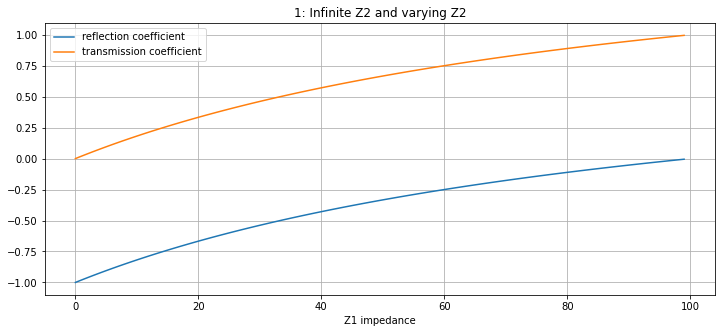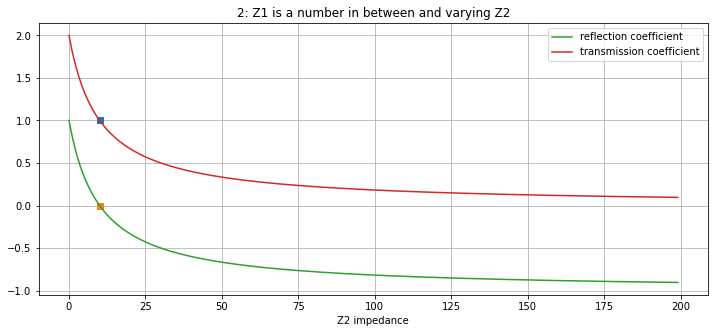# Impedance, Transmission and Reflection#

Waves

In this chapter, impedance, reflection and transmission will be discussed.

import numpy as np
import matplotlib.pyplot as plt


## Impedance#

Impedance is a measure of how difficult it is for a wave to travel through a certain medium.

The physical properties characterizing the model are density ($$\rho$$) and seismic velocity ($$c$$)

The acoustic impedance of each layer is:

$Z = \rho c$

with units $$[kg\,s^{-1}]$$.

Consider a reflectivity model and let’s calculate the impedance for all three layers.def acoustic_impedance(rho, c, layer_number):
z = rho*c
print('The acoustic impedance of layer', layer_number, '=', z, 'kg/s')
return z

rho1 = 2000
v1 = 500
rho2 = 2300
v2 = 1000
rho3 = 2300
v3 = 1500

z1 = acoustic_impedance(rho1, v1, 1)
z2 = acoustic_impedance(rho2, v2, 2)
z3 = acoustic_impedance(rho3, v3, 3)

The acoustic impedance of layer 1 = 1000000 kg/s
The acoustic impedance of layer 2 = 2300000 kg/s
The acoustic impedance of layer 3 = 3450000 kg/s


It can thus be seen that a higher seismic velocity results in a higher impedance and thus easier passage for the wave. This is similar for a higher density.

## Reflection and Transmission#

### Reflection and Transmission (Transverse waves)#

Consider acoustic impedance in a different light where a seismic wave travels through a medium. When an incident wave apporaches a boundary of different density and seismic velocity (thus, different impedance), a wave can reflected or transmitted. We make a few assumptions:

• $$y_i + y_r = y_p$$ (for a continuous string across a boundary and conservation of energy)

• tension in the string must be continuous

Consider the subscript $$i$$ for the incidence wave, $$r$$ for the reflected wave and $$p$$ for the propagating/transmitted wave.

Consider coefficients of reflection and transmission now as the ratio between the amplitude of the reflected/transmitted wave and incident wave respectively.

The reflection coefficient is

$R = \frac{y_{0r}}{y_{0i}}$

and the transmission coefficient is

$P = \frac{y_{0p}}{y_{0i}}$

For this module, we are assuming that the incident wave travels normal to the boundary. Thus $$\theta_i = \theta_r = \theta_p = 0$$.From acoustic impedance, the down-going reflection coefficient for amplitude is given by

$R = \frac{Z_{1}-Z_2}{Z_1+Z_2}$

and the transmission coefficient is

$P = \frac{2Z_{1}}{Z_1+Z_2}$

Considering wave energy transfer, where wave total energy per unit string is defined as $$E = \frac{ \rho \omega^2 {y_0}^2}{2}$$.

$R = \left(\frac{Z_{1}-Z_2}{Z_1+Z_2}\right)^2$
$P = \frac{4 Z_{1} Z_2}{(Z_1+Z_2)^2}$

Note that 1 + R = P always, from conservation of energy

Reflection and transmission coefficients as a function of impedances.

def R_coeff(Z1, Z2):
return (Z1 - Z2)/(Z1 + Z2)

def P_coeff(Z1, Z2):
return (2*Z1)/(Z1 + Z2)

Z1 = list(range(0, 100,1))
Z20 = 100 # substitute for infinity but works too

plt.figure(figsize=(12,5))
plt.xlabel("Z1 impedance")

R_coeffs = [R_coeff(item, Z20) for item in Z1]
P_coeffs = [P_coeff(item, Z20) for item in Z1]

plt.plot(Z1, R_coeffs, label = 'reflection coefficient')
plt.plot(Z1, P_coeffs, label = 'transmission coefficient')
plt.legend()
plt.grid(True)
plt.title("1: Infinite Z2 and varying Z2")
plt.show()plt.figure(figsize=(12,5))
plt.xlabel("Z2 impedance")

Z10 = 10
Z2 = list(range(0, 200,1))

R_coeffs = [R_coeff(Z10, item) for item in Z2]
P_coeffs = [P_coeff(Z10, item) for item in Z2]

x0 = 
y0 = 
y1 = 
plt.plot(x0, y0, "s")
plt.plot(x0, y1, "s")

plt.plot(Z2, R_coeffs, label = 'reflection coefficient')
plt.plot(Z2, P_coeffs, label = 'transmission coefficient')
plt.legend()
plt.grid(True)
plt.title("2: Z1 is a number in between and varying Z2")
plt.show()• Note that when $$Z_1 \to 0$$ (start of graph 1) and $$Z_2 \to \infty$$ (end of graph 2), the reflection coefficient is $$-1$$ and transmission coefficient is $$0$$. This means that the wave is completely reflected and has a phase change of $$\pi$$

• If $$Z_2$$ is zero, the incident wave is reflected, so the reflection coefficient is $$1$$, and the transmission coefficient is $$2$$. No wave can pass through layer 2 if $$Z_2$$ is zero.

• If $$Z_1=Z_2$$ (markers on graph 2), the reflection coefficient is $$0$$ and the transmission coefficient is $$1$$. There is virtually no boundary as the properties, density and seismic velocity, of the media are the same.

### Reflection and Transmission (Longitudinal waves)#

As longitudinal waves travel through a medium, vibrations lead to pressure waves within the medium, with a phase difference of $$\pi$$. Since the longitudinal wave and pressure wave are in anti-phase, the reflection and transmission coefficients for the pressure wave are as follows:

$R = \frac{Z_2-Z_1}{Z_1+Z_2}$
$P = \frac{2Z_2}{Z_1+Z_2}$